﻿ Study of Performance Characteristics of Small Submersible Pump Run as Hydro Turbine Generator

### Study of Performance Characteristics of Small Submersible Pump Run as Hydro Turbine Generator

Kiprop Eliud, Nicholas M. Musembi, Dickson Kindole, Alfred Mukama, Sam K. Kosgei, Yasuyuki Nemoto, Yuichi Nakajo

Sustainable Energy

## Study of Performance Characteristics of Small Submersible Pump Run as Hydro Turbine Generator

Kiprop Eliud1,, Nicholas M. Musembi1, Dickson Kindole1, Alfred Mukama1, Sam K. Kosgei1, Yasuyuki Nemoto2, Yuichi Nakajo2

1Graduate school of Engineering, Ashikaga Institute of Technology, Ashikaga City, Japan

2Division of Renewable Energy and Environment, Ashikaga Institute of Technology, Ashikaga City, Japan

### Abstract

Pumps including submersible types can be used as alternative micro-hydro turbines with minimal technical changes and at a lower cost compared to conventional turbines. However, pump manufacturers do not normally provide the characteristics curves of their pumps working as turbines. Therefore, establishing a relationship between performances of direct (pump) and reverse (turbine) modes is essential in selecting the proper machine for application. For the purpose of realizing the low cost micro hydropower, the authors modified a low cost submersible electric powered pump rated at 250W and converted it to a micro hydro turbine generator. The performance characteristics of this hydro turbine were tested at various conditions of water flow rate, excitation capacitors and load. The input was measured by using flow meter and pressure gauges while the electrical output was measured by using a power analyzer. The maximum electrical output power generated was 207W at water input power of 986.9W and head of 17.97m. The maximum efficiency operating as a pump was 24.57% while on the hydro turbine generator mode it was 22.02%.

• Kiprop Eliud, Nicholas M. Musembi, Dickson Kindole, Alfred Mukama, Sam K. Kosgei, Yasuyuki Nemoto, Yuichi Nakajo. Study of Performance Characteristics of Small Submersible Pump Run as Hydro Turbine Generator. Sustainable Energy. Vol. 5, No. 1, 2017, pp 1-5. http://pubs.sciepub.com/rse/5/1/1
• Eliud, Kiprop, et al. "Study of Performance Characteristics of Small Submersible Pump Run as Hydro Turbine Generator." Sustainable Energy 5.1 (2017): 1-5.
• Eliud, K. , Musembi, N. M. , Kindole, D. , Mukama, A. , Kosgei, S. K. , Nemoto, Y. , & Nakajo, Y. (2017). Study of Performance Characteristics of Small Submersible Pump Run as Hydro Turbine Generator. Sustainable Energy, 5(1), 1-5.
• Eliud, Kiprop, Nicholas M. Musembi, Dickson Kindole, Alfred Mukama, Sam K. Kosgei, Yasuyuki Nemoto, and Yuichi Nakajo. "Study of Performance Characteristics of Small Submersible Pump Run as Hydro Turbine Generator." Sustainable Energy 5, no. 1 (2017): 1-5.

 Import into BibTeX Import into EndNote Import into RefMan Import into RefWorks

1234
Prev Next

### 1. Introduction

Hydropower is among the most widely-used renewable sources of energy. It has little fluctuation which is different from solar and wind power. As a mature technology, hydropower provides over 16% of global electricity production . Large and small scale hydropower can be a tool for rural industrialization and poverty reduction in many developing countries especially in Africa.

The African continent is endowed with enormous hydropower potential that needs to be harnessed. Despite this huge potential, which is enough to meet all the electricity needs of the continent, only a small fraction has been exploited. In general, the large and best sites are developed first on a national scale, so the rate of exploitation of the total generating capacity tends to diminish with time.

As these sites diminish the focus shifts to small and micro hydropower.

In addition, recent studies have shown that electricity generation through small and micro hydropower is gaining ground due to its short project implementation period, minimal environmental impacts and can be located near demand centers.

The key barrier to development of small and micro hydropower has been the lack of appropriate technology and infrastructure in the design and manufacture of turbines, installation and operation. Moreover, currently small and micro hydro turbines are not mass-produced and are generally expensive.

Using pump as turbine (PATs) is an attractive and significant alternative because of the availability, low cost and compact layout. In addition, pumps are relatively simple machines with no special designing and are readily available in most developing countries. Besides, their installation, commissioning and maintenance are easy and cheap. Servicing can also be carried out by pump suppliers, who are usually far numerous than turbine suppliers [2, 3]. From the economical point of view, it is often stated that capital payback period of PATs in the range of 5-500kW is two years or less .

Investigations of various pumps as turbines, suggests that there are several types that are suitable for micro-hydro, running in reverse with and induction generator. These includes; all types of centrifugal pumps, self-priming pumps (only suitable if valve is removed) and dry-motor jacket cooled submersible pumps .

The practical applications of conventional turbines such as Pelton, Francis and Kaplan are well known according to their heads and specific speeds. Due to inadequate experimental data for pumps working as turbines, the field applications of these machines are not yet well defined . Until now, most of research on PATs has been carried out on centrifugal pumps and there has been no much work on other types of pumps as PATs especially submersible pumps. In addition, submersible pumps are cheaper compared to centrifugal pumps. The application of these pumps as PATs can solve the problem of rural electrification in developing countries where affordable small hydropower generation technologies are in high demand.

For the purposes of this research work, the authors modified a low cost submersible electric powered pump with 250W of rated electric power and converted it to a micro turbine generator. First we carried out the test as a normal pump and then reversed and tested as a hydro turbine generator and their respective performance characteristics were compared.

### 2. Basic Theory and Experimental Analysis

When an induction motor machine is connected to an a.c supply, magnetizing current flows from the supply and creates a rotating magnetic field in the machine.

In the case of an induction generator, the magnetizing current, can be supplied in total or in part by capacitors. Since we intended to make an independent induction generator system, the capacitors were the only external source of magnetizing current. The required capacitance was determined by the procedure below.

The no-load apparent power,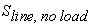of the induction generator is given by;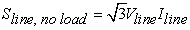(1)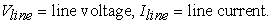Since the no-load apparent power equals the reactive power to be provided by capacitors, the total reactive power,is;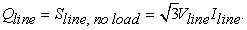(2)

Therefore, the reactive power per phase,is;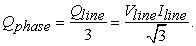(3)

For delta connected capacitors;(4)

Then the phase current,, is given as;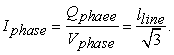(5)

Since the capacitive reactance,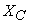is;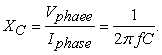(6)

Where;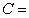Capacitance

Then;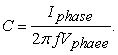(7)

The water power Pw [W] from the pump and into the hydro turbine generator was calculated using the flow rate Q [m3/s] and pressure difference[Pa] between the inlet and outlet and is given by;(8)

The corresponding head was calculated by the pressure difference[Pa], water density ρ [kg/m3] and acceleration due to gravity g [m2/s] and is given as;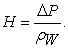(9)

The efficiency of the pumpwas calculated by using output water power[W] and input electrical power[W] and is given as;(10)

The efficiency of the turbine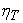was calculated by using output electrical power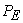[W] and input water power[W] and is given by;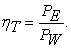(11)

Using the above formulas and the pump specifications in Table 1, the required capacitance to run the above induction motor as induction generator is 15.63μF. It is a good idea to select combination of capacitors that will allow some fine-tuning to be done on site. This can be used to ensure that the operating frequency is acceptable and to maximize the output power . In our current research, we tested using 10, 15 and 20 μF and compared their results.

### 3. Experimental Set up and Methodology

3.1. Pump Test

Figure 1(a) and Figure 1(b) shows photos of the tested pump and its impellers respectively. Figure 2 shows a complete schematic diagram of the experimental set up for pump test. The experimental set up was installed at Ashikaga Institute of Technology, Tochigi Prefecture, Japan. A 250W submersible pump (Super Tepcon Terada PG-250T) was selected for this test. The specifications of the pump are given in Table 1. Flow rate Q and pressure p of water were measured by a vortex flow meter (Universal Flow Monitors UV2M1) and two pressure gauges (Keyence AP-30), respectively. Pressure gauges were installed at the inlet and outlet of the pump. The readings from the flowmeter and the pressure gauges were recorded in a data logger (Hioki LR8431).

The input electrical powerfrom the mains into the pump was measured and recorded by power analyzer (Hioki 3390). The input electrical power was varied by using an inverter from 200W to 600W. To be able to determine the efficiency of the pump a valve was installed at the outlet from the pump and the output was varied from zero to maximum during the experiment. At this time the results of flow rate and electrical input power were measured and stored continuously on data logger and power analyzer respectively. The efficiency of the pump was calculated by using the output water power and input electrical water as shown in equation 11.Download asVeiw figureFigures index
Figure 1. (a) Tested pump (b) Impellers of the tested pumpDownload asVeiw figureFigures index
Figure 2. Experimental set up for pump test

#### Table 1. Specifications of the tested pumpDownload asPowerPoint Slide

Veiw figure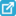View current table in a new window

3.2. Hydro Turbine Generator Test

Figure 3 and Figure 4 respectively shows the complete schematic and a photo of the experimental set up for testing the same 250W submersible pump in the hydro turbine generator mode. On the hydro turbine generator mode, a 1.5kW Ebara pump was used to circulate the water.

As the capacitor for excitation, the film capacitor CMKS-J (for AC250V) was used. The cost of this type of a 10 μF capacitor is less than 10\$. The capacitors were charged from the mains power supply. The load used were bulbs with rated power of between 20W to 140W. The hydro turbine generator output voltage, current, frequency and electrical power were measured by the power analyzer, whereas the input water flow rate was measured by the flow meter. The efficiency of the hydro turbine was calculated by using the electrical output power and the input water power as shown in equation 12.Download asVeiw figureFigures index
Figure 3. Photo of experimental set up

### 4. Experimental Results and Discussion

4.1. Pump Test Results

The results of input electrical power, output water power, head and efficiency of pump test are shown in Figure 5 - Figure 8. The capacitors were charged from the mains power supply. The load used were bulbs with rated power of between 20W to 140W.Download asVeiw figureFigures index
Figure 4. Experimental set up for hydro turbine generator testDownload asVeiw figureFigures index
Figure 5. Input electrical power into the pump

The hydro turbine generator output voltage, current, frequency and electrical power were measured by the power analyzer, whereas the input water flow rate was measured by the flow meter. The efficiency of the hydro turbine was calculated by using the electrical output power and the input water power as shown in equation 12.Download asVeiw figureFigures index
Figure 6. Output water power from the pump

From Figure 5, it is found that the output powerincreases with the increase in the input powerup to an optimum point then it decreases. The maximum output power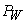was 128W at an input power= 520 W. At this time, the pump efficiency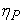was 24.57 % and a head of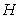= 7.02 m.Download asVeiw figureFigures index
Figure 7. Head generated by the pumpDownload asVeiw figureFigures index
Figure 8. Pump efficiency
4.2. Turbine Test Results

The results of input water power, output electrical power, head and efficiency of the hydro turbine generator test at capacitance of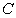= 20 μF are shown in Figure 9 – Figure 12.

The overall maximum output electrical powerwas 207 W at water input power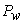= 986.90 W, the capacity of the lamp load= 140 W per phase and capacitance= 20 µF. The voltage, the current per phase, the frequency and head was 113.6 V, 1.05A, 40.021Hz and 17.97m, respectively. At the time of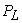= 140 W per phase, the hydro turbine generator efficiency= 20.92%.Download asVeiw figureFigures index
Figure 9. Input water power into the hydro turbine generatorDownload asVeiw figureFigures index
Figure 10. Output electrical power from hydro turbine generatorDownload asVeiw figureFigures index
Figure 11. Required head for generation

For capacitance= 15 µF the maximum output electrical powerwas 196.3 W at water input power= 1004.87 W and lamp load capacity= 100 W per phase. The voltage, the current per phase, frequency, efficiency and head were 134.10 V, 0.8485A, 42.54 Hz, 19.5% and 18.29m respectively. For capacitance= 10 µF the maximum output electrical powerwas 170.3 W at water input power= 1001.72 W and lamp load capacity= 80 W per phase. The voltage, the current per phase, frequency, efficiency and head were 142.49 V, 0.6900A, 49.54 Hz, 17.0% and 19.74m respectively.Download asVeiw figureFigures index
Figure 12. Efficiency of electrical power generation

The highest generation efficiency obtained was 22.02% at capacitance= 15 µF. The voltage, the current per phase, frequency and head were 141.29 V, 0.6835A, 42.02 Hz and 16.6m respectively. This is lower than the pump efficiency by about 2%.

As the capacitance increases, voltage, current and frequency decreases whereas generated electrical power and efficiency increases. In generator operation, the excitation capacitance can be varied depending on the load type and site conditions. The 10 μF capacitance can be used to ensure that the operating frequency is acceptable whereas the 20 μF can be used to maximize the output power. This will be applied depending on the nature of loads used. Where resistive loads only are used 20 μF can be used to obtain maximum output power, whereas, in situations where loads such as motors which are sensitive to operating voltage and frequency are used the 10 μF capacitors can be used to get output power of acceptable quality.

The experimental results show that a submersible turbine can be operated as a hydro turbine generator at various flow rates and heads with little modification and without any technical complications.

These results, for both pump and hydro turbine generator operation modes, agree with the characteristics computed by other researchers [2, 6, 7] where the head and discharge values of turbine mode were found to be higher than in pump mode and the efficiency in hydro turbine mode is lower than efficiency in pump mode. The higher values of flow rates in hydro turbine generator operation indicate larger handling of water as compared to pump operation. Lower efficiency of HTG indicates higher losses in HTG operation mode as compared to pump operation.

### 5. Conclusions

In this study, the authors carried out the performance tests of a submersible pump operating as a pump and then operating as a HTG by using pressure gauges, a flow meter, power analyzer and data logger. It was found that a submersible pump can operate as a HTG at high head and flow rate and lower efficiency compared to pump operation mode. To improve the efficiency of HTG the authors suggests the modification of the impellers and testing again with the new modified impellers. In addition, we think that it is worth carrying out performance test of a submersible pump of bigger capacity and compare the performance with the smaller pump.

### References

  REN21.2014. Renewables 2014 Global Status Report.In article  S. Derakhshan and A. Nourbakhsh, Experimental study of characteristics of curves of centrifugal pumps working as turbines in different specific speeds, Experimental Thermal and Fluid Science, 32(2008). 800-807.In article View Article  J. Furze, Compendium in Small Hydro, University of Aarhus, Faculty of Science, Law and Economics, Ebeltoft Denmark, 2000/2002.In article  A. Nourbakhsh, G. Jahangiri, Inexpensive small hydropower stations for small areas of developing countries, in: Conference on Advanced in Planning-Design and Management of Irrigation Systems as Related to Sustainable Land use, Louvain, Belgium, 1992, pp. 313-319.In article  A. Williams, Pumps as Turbines Users Guide, Intermediate Technology Publications, London, 1995.In article View Article  S. Derakhshan and A. Nourbakhsh, Theoretical, Numerical and Experimental Investigation of Centrifugal Pump in Reverse Operation, Journal of Experimental thermal and Fluid Sciences, 32(2008). 1620-1627.In article  H. Nautiyal, V. Varun, A. Kumar and S. Yadav, Experimental Investigation of Centrifugal Pump Working as Turbine for Small Hydropower Sytems, Journal of Energy Science and Technology, Vol. 1, No. 1 (2011). 79-86.In article  N. Smith, Motors as Generators for Micro Hydro Power, Practical Action, Intermediate Technology Publications, London, 2008.In article